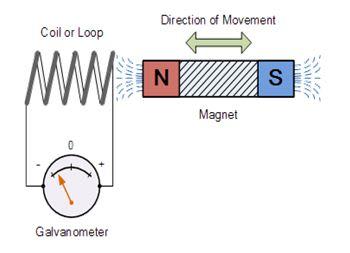# ELECTRO MAGNETIC INDUCTION

What is EMI?

EMI is a phenomenon in which an electric current or electromotive force is induced in the coil due to a magnetic field produced in the coil. Michael Faraday is the scientist behind the invention of electromagnetic induction. Though Faraday was first to publish this but it was discovered autonomously by Joseph Henry. This electromagnetic induction is dependent on Faradays Laws of a magnetic field.

Faraday first proved this electromagnetic theory by winding copper wires to both ends of the iron rod. He explained this theory using a concept of lines of force.

Faraday took a coil and magnet and connected to a galvanometer. So when the galvanometer is connected across the coil the needle in the coil remains at the same position i.e. at the zero position. In this electromagnetic induction phenomenon, an electric current is induced in the coil due to the magnetic field generated by the conductors. When the magnet is held near the coil the electric-field is generated and when the magnet is moved away from the coil there is no deflection in the needle of the galvanometer. The magnetic flux around the coil is directly proportional to the current in the windings of the coil, so more the length of the coil or more the windings more is the emf generated. keeping the magnet in a stationary position and just moving the coil to and fro the magnetic needle in the galvanometer also reflects in that direction. We can infer that when the deflection in the needle is due to the electric-field induced in the coil which is produced by the moving magnet. It can also be noticed that faster the movement of the magnet greater is the voltage or emf produced in the coil.

How can the induced emf be increased?

• By increasing the rate of relative motion between the magnet and the coil. So If the relative speed between the coil and magnet is increased there is more amount of current induced due to constant motion.
• By increasing the turns or windings of the coil. It is such that more the number of turns in the coil more the emf generated.
• By increasing the strength of the magnetic field. More stronger the magnetic field more is the number of lines of force which are responsible for the induction of current.What are the applications of this phenomenon?

• Induction cookers

The induction cooker is the fastest mode used for cooking. This works on electromagnetic induction. In this cooker there is a coil below the vessel which gets heated up due to the mutual induction in the coil, thus this mutual inductance produces a magnetic field which induces emf in the coil.

• AC generators

AC generators work on Faradays laws of induction. It has a coil of wire and rotating and generating the magnetic field. So the electrons move in one direction first and then in the other direction. Thus a generator produces an AC current.

• Transformers

Transformer is another application of Faradays laws of induction and electromagnetic induction. Transformers are mainly used for either increasing or decreasing the magnitude of the alternating current.

• Electromagnetic Flowmeters

These meters are used for measuring the velocity of fluids like blood. When magnetic field is applied to the insulated pipe which ccontains conducting fluids, and the electromotive force is induced due to faraday induction laws. This induced emf is supposed to be directly proportional to the velocity of fluids.